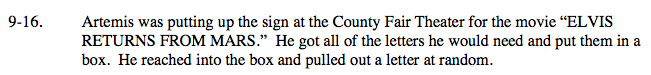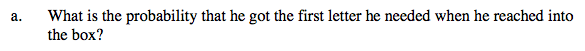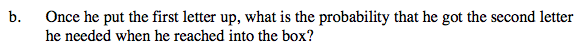### Home > A2C > Chapter Ch9 > Lesson 9.1.1 > Problem9-16

9-16.The first letter he will need is an 'E'. How many E's are there in the message?
How many letters are there total?

$\text{The probability is }\frac{1}{10}.$The next letter is an 'L'. How many L's are there in the message? How many letters are left after he has put up the E?

$\text{The probability is }\frac{1}{19}.$